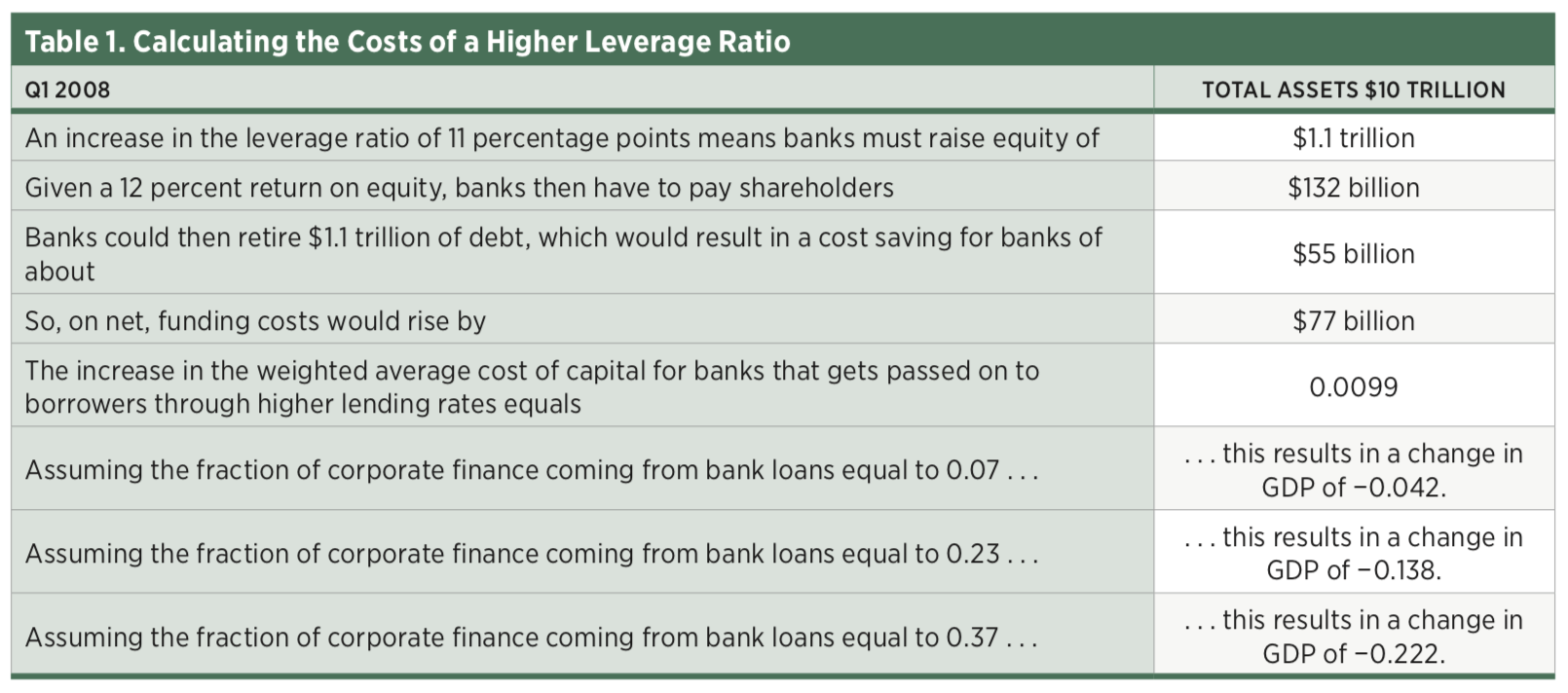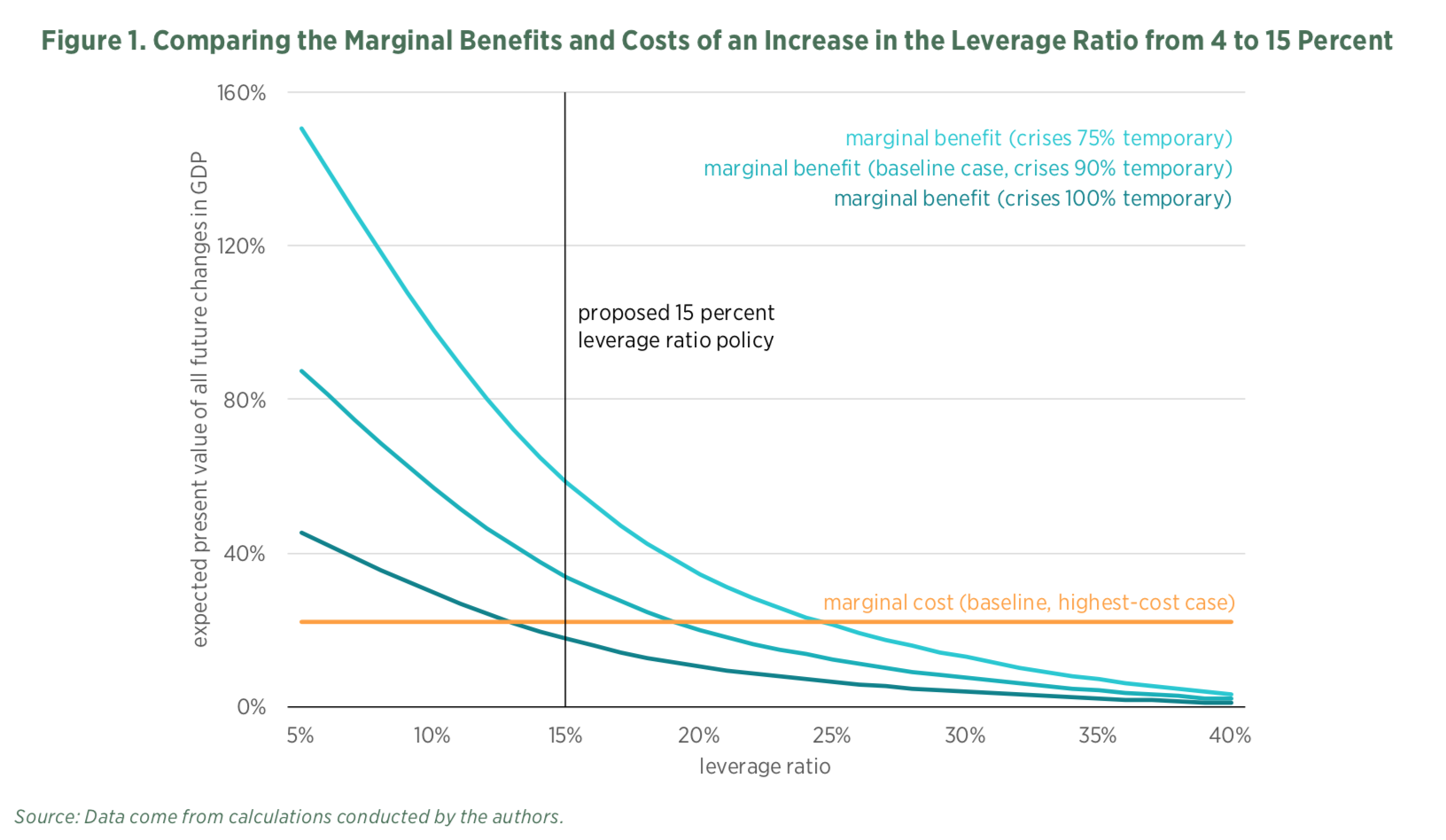April 5, 2018

## Yes, the Benefits of a Higher Leverage Ratio Can Exceed the Costs

Key materials

After the 2007–2008 financial crisis, some academics and lawmakers from both parties have proposed simpler, higher bank capital requirements as a way to strengthen banks and prevent future disasters. For instance, Professors Anat Admati and Martin Hellwig suggest raising the so-called equity-to-asset ratio, or “leverage” ratio, for banks to 20 or 30 percent, while Senators Sherrod Brown and David Vitter cosponsored a bill calling for a ratio of 15 percent. Under such proposals, banks would finance their activities with more equity and less debt.

Two questions arise: How much would a higher bank leverage ratio actually cost? And what benefits would result? We answer these questions in our recent Mercatus Center working paper, which is summarized here.

One plausible answer to the first question, suggested by Franco Modigliani and Merton Miller’s famous theorem, is that raising the leverage ratio has no associated costs. Intuitively, under certain conditions, a bank with a higher leverage ratio would pay a lower return on equity than its more leveraged competitors since the risk of default would be lower. The reduced return on equity, therefore, could fully offset the increased cost of relying more on equity, which pays a relatively higher return than debt.

Critics of the Modigliani-Miller theorem claim that it does not hold and that a higher leverage ratio would raise a bank’s overall cost of funds. The bank would then pass along higher funding costs to borrowers, and the end result would be less borrowing, less capital formation, and a lower GDP. If the critics are right, a higher leverage ratio would be acceptable only if the benefits exceed the costs. Thus, before attempting to reduce the likelihood of a banking crisis, we must justify the approach. We must show that, in decreasing the adverse effect such crises have on GDP, the benefits will exceed any costs that might arise from lower capital formation and lower GDP. We turn to that task now.

### Costs of a Higher Leverage Ratio

To illustrate how a higher leverage ratio could have costs that translate into forgone GDP, consider the example summarized in table 1. In the first quarter of 2008, total assets for US banks in our sample equaled about \$10 trillion. An 11 percentage point increase—equal to the increase in the leverage ratio from 4 percent to 15 percent that we consider in the paper—would require the banks to raise an additional \$1.1 trillion in equity while retiring \$1.1 trillion in debt.In our highest-cost case, assuming the return on equity equals 12 percent, banks would have to pay an additional \$132 billion to shareholders if the leverage ratio rises by 11 percentage points. The increased payments to shareholders would be partially offset by lower interest expenses from retiring the debt. Assuming a 5 percent rate of interest, the reduced debt costs would amount to \$55 billion for an 11 percentage point increase in the leverage ratio.

On net, banks with total assets equaling \$10 trillion would face additional costs of capital of \$77 billion for an 11 percentage point increase. Looked at another way, for an 11 percentage point higher leverage ratio, the costs would rise by 99 basis points. Banks might try to recover these costs by charging higher interest rates on their loans, which could translate into less capital formation and thus forgone GDP.

To calculate forgone GDP, one important factor is the degree to which companies rely on banks for funding. In the United States, recent data suggest that bank lending, as a fraction of all corporate funding, averages about 7 percent. If, however, the higher costs in bank lending spill over to other debt markets, then it may be useful to consider not just bank loans but also total debt as a fraction of corporate funding. Accordingly, we use a debt-to-capital ratio of 37 percent in our baseline case and 23 percent as an alternative.

The results show a range of output declines. For a leverage ratio that is 11 percentage points higher, assuming a 7 percent fraction of corporate funding coming from bank loans, output declines by 4.2 percent. Assuming instead a debt-to-capital ratio of 23 percent, output declines by 13.8 percent. And assuming a debt-to-capital ratio of 37 percent, output declines by 22.2 percent.

### Benefits of a Higher Leverage Ratio

One approach to estimating the benefits of a higher leverage ratio is to estimate the relationship between the leverage ratio and the probability of a crisis and then estimate the effects of crises on the rate of economic growth. On the first estimate, we find empirically that the probability of a banking crisis varies inversely with the leverage ratio, so that a higher leverage ratio produces benefits in the form of lower crisis costs.

On the second estimate, the Bank of England’s 2010 Financial Stability Report and Miles, Yang, and Marcheggiano assume that the cost of a crisis equals a 10 percent decline in GDP and that 75 percent of the effects of a crisis are temporary, lasting five years. For the United States, in our baseline case we assume 90 percent of crisis effects are temporary and last two years. We also estimate that for the United States the cost of a crisis equals a 10.3 percent decline in GDP. Additionally, we examine the impact of assuming crises’ effects are either 75 percent temporary or 100 percent temporary. The expected benefit of higher capital requirements per percentage point reduction in the probability of a crisis equals

• 13.3 percent of one year’s GDP if crises have 75 percent temporary effects,
• 7.7 percent of one year’s GDP if crises have 90 percent temporary effects, or
• 4 percent of one year’s GDP if crises have only temporary effects.

### Comparing the Benefits and Costs

Figure 1 depicts the marginal benefits and marginal costs of changing the leverage ratio from 4 percent (the Basel III US leverage ratio in 2014) to 15 percent. Under the highest cost assumption, which implies that output would decline by 22.2 percent, the marginal benefits of increasing the leverage ratio to 15 percent exceeds the marginal costs when crises have some permanent effects. If crises have only 75 percent temporary effects the benefits of increasing the leverage ratio to 24 percent would roughly equal the costs. If crises have only 90 percent temporary effects the benefits of increasing the leverage ratio to 19 percent would roughly equal the costs. When we assume costs are at their highest and crises have only temporary effects, the marginal costs of raising the leverage ratio to 15 percent exceed the marginal benefits; in that case, the leverage ratio that equates marginal costs and benefits equals 12 percent.### Conclusion

We find that increasing bank capital through a higher leverage ratio can help reduce the probability of banking crises and thus prevent the harmful effects that crises exert on the economy. These benefits are high enough to offset the costs of raising the equity-to-assets ratio for banks. They exceed the costs for lower-cost scenarios, but even if we assume that the costs of raising the ratio to 15 percent are high, the marginal benefits can cover the marginal costs.